图像阈值分割_图像阈值分割python - CSDN
• 1、Otsu阈值分割 2、自适应阈值分割 3、 最大熵阈值分割法 4、 迭代阈值分割  5、测验   1、Otsu阈值分割  Otsu（大津法或最大类间方差法）使用的是聚类的思想，把图像的灰度数按灰度级分成2个部分，...
目录

1、Otsu阈值分割

2、自适应阈值分割

3、 最大熵阈值分割法

4、 迭代阈值分割

5、测验

1、Otsu阈值分割

Otsu（大津法或最大类间方差法）使用的是聚类的思想，把图像的灰度数按灰度级分成2个部分，使得两个部分之间的灰度值差异最大，每个部分之间的灰度差异最小，通过方差的计算来寻找一个合适的灰度级别来划分。 所以可以在二值化的时候采用otsu算法来自动选取阈值进行二值化。otsu算法被认为是图像分割中阈值选取的最佳算法，计算简单，不受图像亮度和对比度的影响。因此，使类间方差最大的分割意味着错分概率最小。

参考链接：https://www.cnblogs.com/moon1992/p/5092726.html

opencv调用格式为：threshold(sourceImage, dstImage, 0, 255, CV_THRESH_OTSU);

sourceImage：输入图像；

dstImage：输出图像；

otsu算法的源码如下：

Mat OtsuAlgThreshold(Mat &image)
{
if (image.channels() != 1)
{
cout << "Please input Gray-image!" << endl;
}
int T = 0; //Otsu算法阈值
double varValue = 0; //类间方差中间值保存
double w0 = 0; //前景像素点数所占比例
double w1 = 0; //背景像素点数所占比例
double u0 = 0; //前景平均灰度
double u1 = 0; //背景平均灰度
double Histogram = { 0 }; //灰度直方图，下标是灰度值，保存内容是灰度值对应的像素点总数
uchar *data = image.data;

double totalNum = image.rows*image.cols; //像素总数

for (int i = 0; i < image.rows; i++)
{
for (int j = 0; j < image.cols; j++)
{
if (image.at<uchar>(i, j) != 0) Histogram[data[i*image.step + j]]++;
}
}
int minpos, maxpos;
for (int i = 0; i < 255; i++)
{
if (Histogram[i] != 0)
{
minpos = i;
break;
}
}
for (int i = 255; i > 0; i--)
{
if (Histogram[i] != 0)
{
maxpos = i;
break;
}
}

for (int i = minpos; i <= maxpos; i++)
{
//每次遍历之前初始化各变量
w1 = 0;       u1 = 0;       w0 = 0;       u0 = 0;
//***********背景各分量值计算**************************
for (int j = 0; j <= i; j++) //背景部分各值计算
{
w1 += Histogram[j];   //背景部分像素点总数
u1 += j*Histogram[j]; //背景部分像素总灰度和
}
if (w1 == 0) //背景部分像素点数为0时退出
{
break;
}
u1 = u1 / w1; //背景像素平均灰度
w1 = w1 / totalNum; // 背景部分像素点数所占比例
//***********背景各分量值计算**************************

//***********前景各分量值计算**************************
for (int k = i + 1; k < 255; k++)
{
w0 += Histogram[k];  //前景部分像素点总数
u0 += k*Histogram[k]; //前景部分像素总灰度和
}
if (w0 == 0) //前景部分像素点数为0时退出
{
break;
}
u0 = u0 / w0; //前景像素平均灰度
w0 = w0 / totalNum; // 前景部分像素点数所占比例
//***********前景各分量值计算**************************

//***********类间方差计算******************************
double varValueI = w0*w1*(u1 - u0)*(u1 - u0); //当前类间方差计算
if (varValue < varValueI)
{
varValue = varValueI;
T = i;
}
}
Mat dst;
threshold(image, dst, T, 255, CV_THRESH_OTSU);
return dst;
}

2、自适应阈值分割

二值化算法是用输入像素的值I与一个值C来比较，根据比较结果确定输出值。

自适应二值化的每一个像素的比较值C都不同，比较值C由这个像素为中心的一个块范围计算在减去差值delta得到。

其中，C的常用计算方法有两种：

a、平均值减去差值delta（使用盒过滤boxfilter，性能会非常不错）

b、高斯分布加权和减去差值delta (使用高斯滤波GaussionBlur)

void myadaptive(InputArray _src, OutputArray _dst, double maxValue,
int method, int type, int blockSize, double delta)
{
Mat src = _src.getMat();

CV_Assert(src.type() == CV_8UC1);
CV_Assert(blockSize % 2 == 1 && blockSize > 1);
Size size = src.size();

_dst.create(size, src.type());
Mat dst = _dst.getMat();

if (maxValue < 0)
{
dst = Scalar(0);
return;
}

Mat mean;
if (src.data != dst.data)
mean = dst;
{
GaussianBlur(src, mean, Size(blockSize, blockSize), 0, 0, BORDER_REPLICATE);
}
{
boxFilter(src, mean, src.type(), Size(blockSize, blockSize),
Point(-1, -1), true, BORDER_REPLICATE);
}
else
{
}

int i, j;
uchar imaxval = saturate_cast<uchar>(maxValue);
int idelta = type == THRESH_BINARY ? cvCeil(delta) : cvFloor(delta);
uchar tab;

if (type == CV_THRESH_BINARY)
for (i = 0; i < 768; i++)
tab[i] = (uchar)(i - 255 > -idelta ? imaxval : 0);
else if (type == CV_THRESH_BINARY_INV)
for (i = 0; i < 768; i++)
tab[i] = (uchar)(i - 255 <= -idelta ? imaxval : 0);
else
{
}

if (src.isContinuous() && mean.isContinuous() && dst.isContinuous())
{
size.width *= size.height;
size.height = 1;
}

for (i = 0; i < size.height; i++)
{
const uchar* sdata = src.data + src.step*i;
const uchar* mdata = mean.data + mean.step*i;
uchar* ddata = dst.data + dst.step*i;

for (j = 0; j < size.width; j++)
// 将[-255, 255] 映射到[0, 510]然后查表
ddata[j] = tab[sdata[j] - mdata[j] + 255];
}
}

3、 最大熵阈值分割法

参考链接：https://blog.csdn.net/qq_27668313/article/details/77949596

https://blog.csdn.net/robin__chou/article/details/53931442

https://blog.csdn.net/xw20084898/article/details/22760169

最大熵阈值分割函数源码如下：

Mat EntropySeg(Mat src)
{
int tbHist = { 0 };
int index = 0;
double Property = 0.0;
double maxEntropy = -1.0;
double frontEntropy = 0.0;
double backEntropy = 0.0;
int TotalPixel = 0;
int nCol = src.cols*src.channels();
for (int i = 0; i < src.rows; i++)
{
uchar* pData = src.ptr<uchar>(i);
for (int j = 0; j < nCol; j++)
{
++TotalPixel;
tbHist[pData[j]] += 1;
}
}

for (int i = 0; i < 256; i++)
{
double backTotal = 0;
for (int j = 0; j < i; j++)
{
backTotal += tbHist[j];
}

for (int j = 0; j < i; j++)
{
if (tbHist[j] != 0)
{
Property = tbHist[j] / backTotal;
backEntropy += -Property*logf((float)Property);
}
}

for (int k = i; k < 256; k++)
{
if (tbHist[k] != 0)
{
Property = tbHist[k] / (TotalPixel - backTotal);
frontEntropy += -Property * logf((float)Property);
}
}

if (frontEntropy + backEntropy > maxEntropy)
{
maxEntropy = frontEntropy + backEntropy;
index = i;
}

frontEntropy = 0.0;
backEntropy = 0.0;
}

Mat dst;
threshold(src, dst, index, 255, 0);
return dst;
}

4、 迭代阈值分割

通过迭代方法选择阈值， 计算方法如下：

（1）选择灰度图的平均值作为初始阈值T0 ;

（2）计算小于等于T0的平均值T1, 和大于T0的平均值T2;

（3）新的阈值为T = （T1 + T2）/ 2;

（4）比较T和T0,若相等，则返回T，即为迭代阈值; 否则 T0 = T,重复（1）-（3）

迭代阈值分割的源码如下：

Mat IterationThreshold(Mat src)
{
int width = src.cols;
int height = src.rows;
int hisData = { 0 };
for (int j = 0; j < height; j++)
{
uchar* data = src.ptr<uchar>(j);
for (int i = 0; i < width; i++)
hisData[data[i]]++;
}

int T0 = 0;
for (int i = 0; i < 256; i++)
{
T0 += i*hisData[i];
}
T0 /= width*height;

int T1 = 0, T2 = 0;
int num1 = 0, num2 = 0;
int T = 0;
while (1)
{
for (int i = 0; i < T0 + 1; i++)
{
T1 += i*hisData[i];
num1 += hisData[i];
}
if (num1 == 0)
continue;
for (int i = T0 + 1; i < 256; i++)
{
T2 += i*hisData[i];
num2 += hisData[i];
}
if (num2 == 0)
continue;

T = (T1 / num1 + T2 / num2) / 2;

if (T == T0)
break;
else
T0 = T;
}

Mat dst;
threshold(src, dst, T, 255, 0);
return dst;
}

5、测验

void main()
{
cvtColor(src, src, COLOR_RGB2GRAY);

Mat bw1, bw2, bw3, bw4;
bw2 = EntropySeg(src);
bw3 = OtsuAlgThreshold(src);
bw4 = IterationThreshold(src);

imshow("source", src);
imshow("自适应阈值分割", bw1);
imshow("最大熵阈值分割", bw2);
imshow("Otsu阈值分割", bw3);
imshow("迭代阈值分割", bw4);
waitKey(0);
}

测验结果：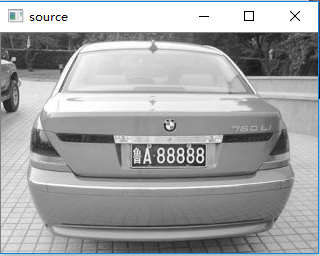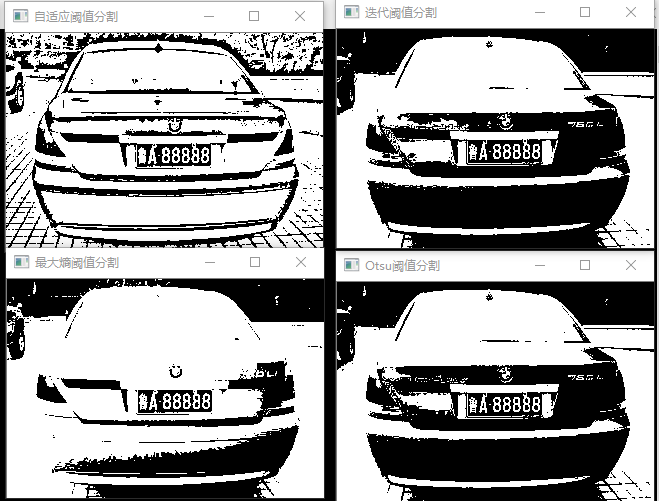展开全文opencv
• 用于实现灰度图像阈值分割的二维最大熵方法，使用matlab实现图像阈值分割
• 基于matlab的图像阈值分割算法，重点研究了最大熵法、迭代法、类间类内方差比法。并且附有源代码。阈值分割
• 图像的阈值处理 一幅图像包括目标物体、背景还有噪声，要想从多值的数字图像中直接提取... 阈值分割法的特点是:适用于目标与背景灰度有较强对比的情况，重要的是背景或物体的灰度比较单一，而且总可以得到封闭且连...
图像的阈值处理      一幅图像包括目标物体、背景还有噪声，要想从多值的数字图像中直接提取出目标物体，常用的方法就是设定一个阈值T，用T将图像的数据分成两部分：大于T的像素群和小于T的像素群。这是研究灰度变换的最特殊的方法，称为图像的二值化（Binarization）。       阈值分割法的特点是:适用于目标与背景灰度有较强对比的情况，重要的是背景或物体的灰度比较单一，而且总可以得到封闭且连通区域的边界。（一）简单阈值选取一个全局阈值，然后就把整幅图像分成非黑即白的二值图像。函数为cv2.threshold( )这个函数有四个参数，第一个是原图像矩阵，第二个是进行分类的阈值，第三个是高于（低于）阈值时赋予的新值，第四个是一个方法选择参数，常用的有：cv2.THRESH_BINARY（黑白二值）cv2.THRESH_BINARY_INV（黑白二值翻转）cv2.THRESH_TRUNC（得到额图像为多像素值）cv2.THRESH_TOZERO（当像素高于阈值时像素设置为自己提供的像素值，低于阈值时不作处理）cv2.THRESH_TOZERO_INV（当像素低于阈值时设置为自己提供的像素值，高于阈值时不作处理）这个函数返回两个值，第一个值为阈值，第二个就是阈值处理后的图像矩阵。img = cv2.imread('4.jpg', 0)
ret, thresh1 = cv2.threshold(img, 127, 255, cv2.THRESH_BINARY)  # binary （黑白二值）
ret, thresh2 = cv2.threshold(img, 127, 255, cv2.THRESH_BINARY_INV)  # （黑白二值反转）
ret, thresh3 = cv2.threshold(img, 127, 255, cv2.THRESH_TRUNC)  # 得到的图像为多像素值
ret, thresh4 = cv2.threshold(img, 127, 255, cv2.THRESH_TOZERO)  # 高于阈值时像素设置为255，低于阈值时不作处理
ret, thresh5 = cv2.threshold(img, 127, 255, cv2.THRESH_TOZERO_INV)  # 低于阈值时设置为255，高于阈值时不作处理

print(ret)

cv2.imshow('thresh1', thresh1)
cv2.imshow('thresh2', thresh2)
cv2.imshow('thresh3', thresh3)
cv2.imshow('thresh4', thresh4)
cv2.imshow('thresh5', thresh5)
cv2.imshow('grey-map', img)
cv2.waitKey(0)
cv2.destroyAllWindows()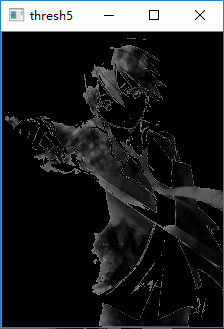（二）自适应阈值一中的简单阈值是一种全局性的阈值，只需要设定一个阈值，整个图像都和这个阈值比较。而自适应阈值可以看成一种局部性的阈值，通过设定一个区域大小，比较这个点与区域大小里面像素点 的平均值（或者其他特征）的大小关系确定这个像素点的情况。使用的函数为：# 第一个参数为原始图像矩阵，第二个参数为像素值上限，第三个是自适应方法（adaptive method）：#                                              -----cv2.ADAPTIVE_THRESH_MEAN_C:领域内均值#                                              -----cv2.ADAPTIVE_THRESH_GAUSSIAN_C:领域内像素点加权和，权重为一个高斯窗口# 第四个值的赋值方法：只有cv2.THRESH_BINARY和cv2.THRESH_BINARY_INV# 第五个Block size：设定领域大小（一个正方形的领域）# 第六个参数C，阈值等于均值或者加权值减去这个常数（为0相当于阈值，就是求得领域内均值或者加权值）# 这种方法理论上得到的效果更好，相当于在动态自适应的调整属于自己像素点的阈值，而不是整幅图都用一个阈值img = cv2.imread('4.jpg', 0)
ret, th1 = cv2.threshold(img, 127, 255, cv2.THRESH_BINARY)
# 第四个值的赋值方法：只有cv2.THRESH_BINARY和cv2.THRESH_BINARY_INV
# 第五个Block size：设定领域大小（一个正方形的领域）
# 第六个参数C，阈值等于均值或者加权值减去这个常数（为0相当于阈值，就是求得领域内均值或者加权值）
# 这种方法理论上得到的效果更好，相当于在动态自适应的调整属于自己像素点的阈值，而不是整幅图都用一个阈值
cv2.imshow('img', img)
cv2.imshow('th1', th1)
cv2.imshow('th2', th2)
cv2.imshow('th3', th3)
cv2.imshow('th4', th4)
cv2.waitKey(0)
cv2.destroyAllWindows()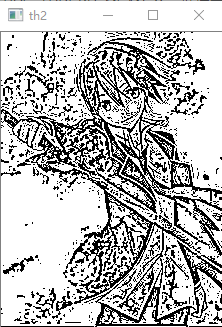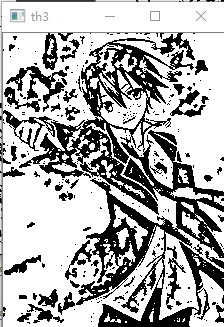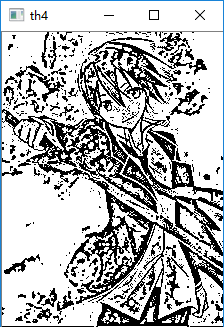对于第五个参数的窗口越来越小时，发现得到的图像越来越细了，可以设想，如果把窗口设置的足够大的话（不能超过图像大小），那么得到的结果可能就和第二幅图像的相同了。（三）Otsu's二值化cv2.threshold( )函数有两个返回值，一个是阈值，第二个是处理后的图像矩阵。前面对于阈值的设定上，我们选择的阈值都是127，在实际情况中，有的图像阈值不是127得到的图像效果更好。那么这里就需要算法自己去寻找一个阈值，而Otsu's就可以自己找到一个认为最好的阈值。并且Otsu's非常适合于图像灰度直方图(只有灰度图像才有)具有双峰的情况。他会在双峰之间找到一个值作为阈值，对于非双峰图像，可能并不是很好用。那么经过Otsu's得到的那个阈值就是函数cv2.threshold的第一个参数了。因为Otsu's方法会产生一个阈值，那么函数cv2.threshold( )的第二个参数（设定阈值）就是0了，并且在cv2.threshold的方法参数中还得加上语句cv2.THRESH_OTSU.在下列这些程序和图片中大家会有鲜明的体会：img = cv2.imread('2.jpg', 0)
ret1, th1 = cv2.threshold(img, 127, 255, cv2.THRESH_BINARY)  # 简单滤波
ret2, th2 = cv2.threshold(img, 0, 255, cv2.THRESH_BINARY+cv2.THRESH_OTSU)  # Otsu 滤波
print(ret2)
cv2.imshow('img', img)
cv2.imshow('th1', th1)
cv2.imshow('th2', th2)
# 用于解决matplotlib中显示图像的中文乱码问题
plt.rcParams['font.sans-serif'] = ['SimHei']
plt.rcParams['axes.unicode_minus'] = False
plt.hist(img.ravel(), 256)
plt.title('灰度直方图')
plt.show()
cv2.waitKey(0)
cv2.destroyAllWindows()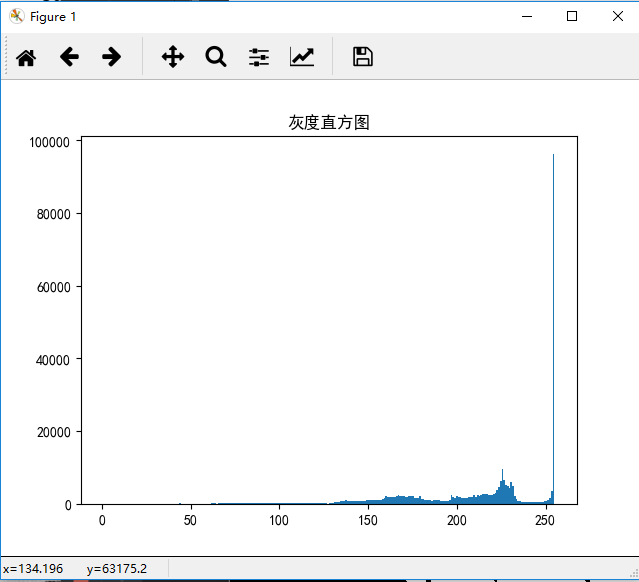展开全文• 一、图像分割 图像分割就是将图像分为一些有意义的区域，然后可以对这些区域进行描述，相当于提取出某些目标区域的特征。图像分割的基础是像素间的相似性和跳变性。 通常，我们对图像进行分割分割的小区域是某种...
一、图像分割
图像分割就是将图像分为一些有意义的区域，然后可以对这些区域进行描述，相当于提取出某些目标区域的特征。图像分割的基础是像素间的相似性和跳变性。
通常，我们对图像进行分割，分割的小区域是某种意义下具有共同属性的像素的连通集合。各区域类某种性质，如灰度、纹理有相似性。区域内部是连通，无过多小孔。并且有明显边界。
图像分割的四种不同的基本方法

边缘检测：检测出边缘，再将边缘像素连接，构成边界形成分割。找出目标物理的轮廓，进行目标的分析、识别、测量
阈值分割：最常用法。有直方图门限选择，半阈值选择图像分割，迭代阈值。
边界方法：直接确定取余边界，实现分割；有边界跟踪法，轮廓提取法。
区域法：将各个像素划归到相应物体或者区域的像素聚类法

图像的阈值分割
有以下三种基本方法

直方图门限选择
半阈值选择图像分割
迭代阈值图像分割

第一种直方图门限选择确定阈值T的原理主要是根据，物理的背景和前景往往是不通，体现在直方图上的化就是其灰度值成明显的双峰值。
例：下面有一幅图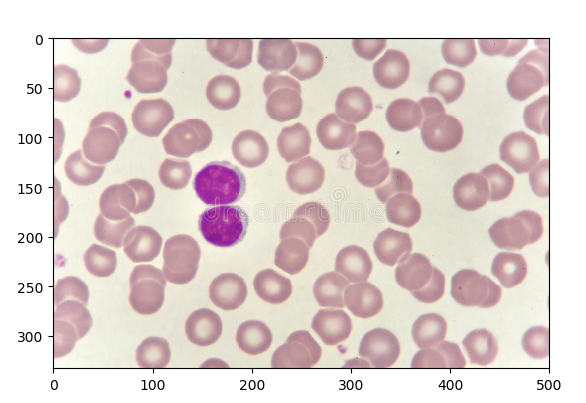其对应的，灰度级直方图如下：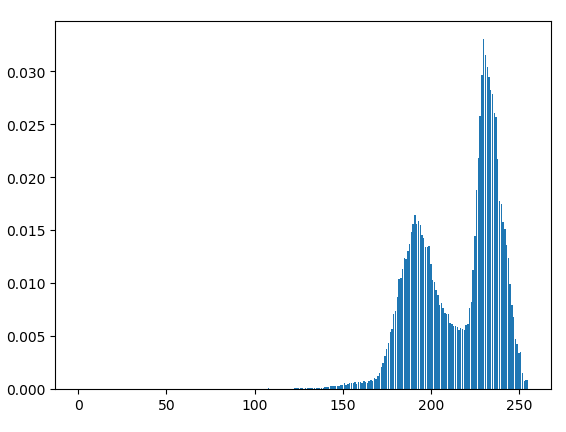我们可以很清晰的看到，波谷大概位于215处为止。现在我们对此进行阈值分割，其效果如下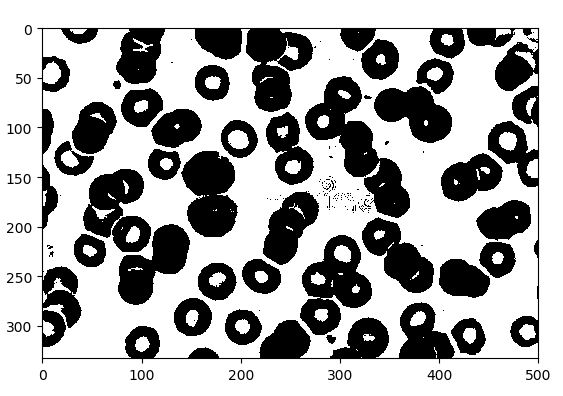那么，我们怎么选择对应的波谷呢？如果有多个波谷、波峰该怎么办呢？
最简单粗暴的就是通过人工来填写阈值。当然这种方法也就违背了我们做图像识别的意义了。
如果有多个波谷，那么我们选择多个阈值即可。其数学表达式大概如下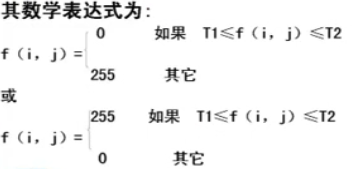半阈值选择分割法
该方法原理就是，不论图像的直方图具有双峰还是多峰，阈值化之后原多值图像都将会变成二值图像。如果希望只将图像的背景变成二值图像，而物理任然为多值图像，此时，我们可以采用半阈值分割法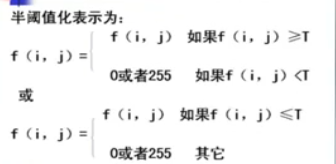半阈值分割法的效果图如下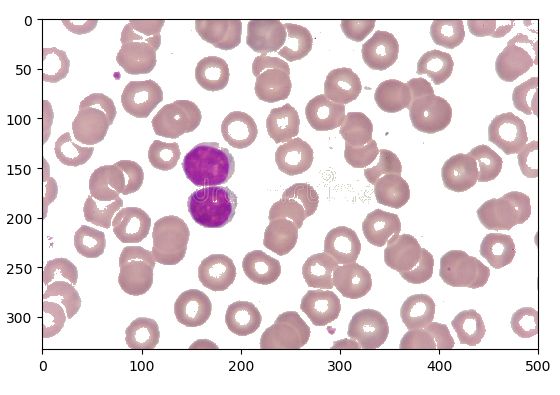迭代阈值分割法
迭代阈值分割法是一种自动算出阈值的，不需要人工参与的。
其基本思想，选择一个阈值作为初值，按照某种策略，直到满足某种条件，跳出循环，输出阈值。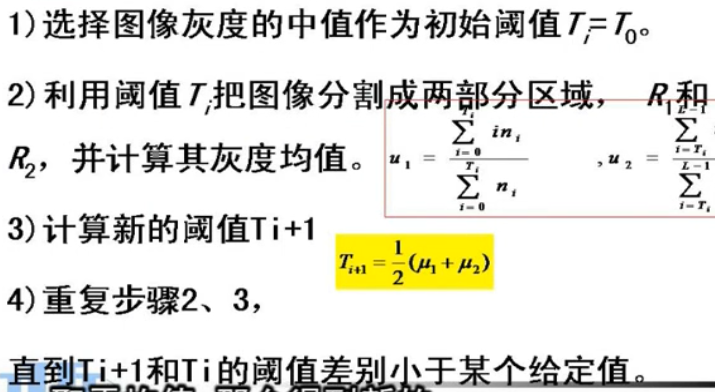用代码实现，即如下
    # 自动迭代阈值法
def  GetThresholdvalue(self):
# 辅助函数，得到图像的统计数据
def helper1():
static = np.array([0 for i in range(0, 256)])
s = np.array([])
for y in range(0,len(self.Img)):
for x in range(0,len(self.Img[y])):
Col=self.Img[y][x]
static[Col]=static[Col]+1
return static
# 下面几步是用来确定初始化阈值的
def helper2(arr,pos):
sum=0
c=0
for i in  range(0,len(arr)):
sum=sum+arr[i]*(i+pos)
c = c + arr[i]
if c==0:
return 0
return int(sum/c)
tv = helper1() #得到图像的统计数据
c_init=helper2(tv,0) # 计算出平均灰度值，作为初始阈值
# 下面开始迭代
l_tv=tv[:c_init]
r_tv=tv[c_init:]
lastavg=c_init
while True:
L_avg=helper2(l_tv,pos=0)
R_avg=helper2(r_tv,pos=lastavg)
avg=int((L_avg+R_avg)/2)
if np.abs(avg-lastavg)<2:
break
l_tv = tv[:avg]
r_tv = tv[avg:]
lastavg=avg
return lastavg



展开全文计算机视觉 opencv
• 图像分割是模式识别和计算机视觉中很重要的
        图像分割是模式识别和计算机视觉中很重要的一个部分，基于阈值的图像分割具有简单、计算量小、效率高等特点，在实际图像处理中具有广泛的应用。经过国内外学者的广泛努力，已经提出了数以百计的阈值分割的算法，依据阈值分割算法本身所具有的准则或特性，可以分为迭代法、最大类间误差法（Otsu）、最大熵法等。本文主要介绍以上几种阈值分割方法。
（一）迭代法
迭代法是一种比较简单的阈值分割方法，其思想：设置阈值的初始值为图像灰度最大值和最小值的平均，根据阈值划分图像为目标和背景，并分别将其灰度值求和，计算目标和背景的平均灰度，并判断阈值是否等于目标和背景平均灰度的和的平均，若相等，则阈值即为其平均，否则，将阈值设置为目标和背景灰度平局值的和的一半，继续迭代，直至计算出阈值。
算法过程：
1、计算图像的最大灰度值Ｚmax、最小灰度值Ｚmin，求平均得到初始阈值ｔｋ。
zmax=max(max(I));zmin=min(min(I));
tk=(zmax+zmin)/2;
2、根据阈值划分图像为目标和背景，并分别计算其灰度均值
z1=foregroundsum/iforeground;
z2=foregroundsum/ibackground;
tktmp=uint8((z1+z2)/2);
3、判断tk是否等于tktmp，若相等则退出循环，阈值为tktmp，否则，将tktmp赋给tk，转2继续执行迭代。
参考代码：

close all;%关闭所有窗口
clear;%清除变量的状态数据
clc;%清除命令行

subplot(1,2,1);
imshow(I);
title('1 rice的原图');

%迭代式阈值分割
zmax=max(max(I));%取出最大灰度值
zmin=min(min(I));%取出最小灰度值
tk=(zmax+zmin)/2;
bcal=1;
[m,n]=size(I);
while(bcal)
%定义前景和背景数
iforeground=0;
ibackground=0;
%定义前景和背景灰度总和
foregroundsum=0;
backgroundsum=0;
for i=1:m
for j=1:n
tmp=I(i,j);
if(tmp>=tk)
%前景灰度值
iforeground=iforeground+1;
foregroundsum=foregroundsum+double(tmp);
else
ibackground=ibackground+1;
backgroundsum=backgroundsum+double(tmp);
end
end
end
%计算前景和背景的平均值
z1=foregroundsum/iforeground;
z2=foregroundsum/ibackground;
tktmp=uint8((z1+z2)/2);
if(tktmp==tk)
bcal=0;
else
tk=tktmp;
end
%当阈值不再变化时,说明迭代结束
end
disp(strcat('迭代的阈值:',num2str(tk)));%在command window里显示出 :迭代的阈值:阈值
newI=im2bw(I,double(tk)/255);%函数im2bw使用阈值（threshold）变换法把灰度图像（grayscale image）(二)最大类间误差法（Otus）

大津算法是1979年日本学者大津在文章A Tlreshold Selection Method from Gray-Level Histograms中提出的自适应的阈值确定的方法，简称ＯＴＳＵ。其思想：根据灰度特性，将图像分为目标和背景２部分，目标和背景之间的类间差越大，说明构成图像的２部分的差别越大，因此类间方差最大的分割即意味着错分概率最小，计算以每个灰度值为阈值的分割的类间方差，其中类间方差最大的值即为阈值。
算法过程：
1、计算每个灰度值的概率并计算目标和背景的分布概率以及平均灰度值和方差。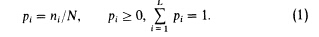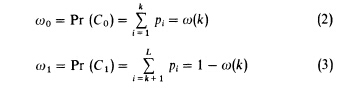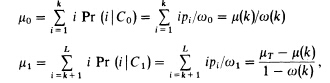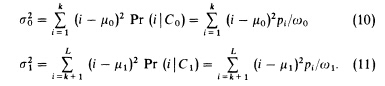2、计算类间差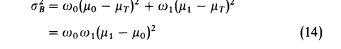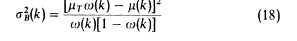3、取类间差最大的灰度值即为阈值参考代码：
Matlab实现了大津算法。
close all;%关闭所有窗口
clear;%清除变量的状态数据
clc;%清除命令行
subplot(1,2,1);
imshow(I);
title('1 rice的原图');
bw=graythresh(I);
disp(strcat('otsu阈值分割的阈值:',num2str(bw*255)));%在command window里显示出 :迭代的阈值:阈值
newII=im2bw(I,bw);
subplot(1,2,2);
imshow(newII);
title('2 rice的otsu阈值分割');
(三)、最小误差法
最小误差法是KITTLER1984年在MINIMUM ERROR THRESHOLDING文章中提出的一种基于直方图的阈值分割方法，简称KITTLER算法。其思想：假设灰度图像由目标和背景组成，且目标和背景满足一混合高斯分布，计算目标和背景的均值、方差，根据最小分类误差思想得到的最小误差目标函数，取目标函数最小时的阈值即为最佳阈值。按此阈值将图像分割为二值图像。
算法过程：
1、计算目标和背景的均值、方差。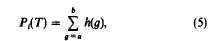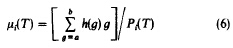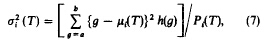2、根据最小分类误差思想得到最小误差目标函数。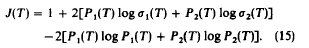3、取使目标函数最小值为阈值。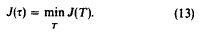参考代码：
I = imread('rice.bmp');
MAXD = 100000;
imag = imag(:,:,1);
[counts, x] = imhist(imag);  % counts are the histogram. x is the intensity level.
GradeI = length(x);   % the resolusion of the intensity. i.e. 256 for uint8.
J_t = zeros(GradeI, 1);  % criterion function
prob = counts ./ sum(counts);  % Probability distribution
meanT = x' * prob;  % Total mean level of the picture
% Initialization
w0 = prob(1);   % Probability of the first class
miuK = 0;   % First-order cumulative moments of the histogram up to the kth level.
J_t(1) = MAXD;
for i = 1 : n
w0 = w0 + prob(i+1);
miuK = miuK + i * prob(i+1);  % first-order cumulative moment
if (w0 == 0) || (w0 == 1)
J_t(i+1) = MAXD;    % T = i
else
miu1 = miuK / w0;
miu2 = (meanT-miuK) / (1-w0);
var1 = (((0 : i)'-miu1).^2)' * prob(1 : i+1);
var1 = var1 / w0;  % variance
var2 = (((i+1 : n)'-miu2).^2)' * prob(i+2 : n+1);
var2 = var2 / (1-w0);
if var1 > 0 && var2 > 0   % in case of var1=0 or var2 =0
J_t(i+1) = 1+2*w0 * log(var1)+2*(1-w0) * log(var2)-2*w0*log(w0)-2*(1-w0)*log(1-w0);
else
J_t(i+1) = MAXD;
end
end
end
minJ = min(J_t);
index = find(J_t == minJ);
th = mean(index);
th = (th-1)/n
imagBW = im2bw(imag, th);
figure, imshow(I_bw);


展开全文• 图像分割就是把图像中具有特殊涵义的不同区域分开来，这些区域是互不相交的，每个区域都满足特定区域的一致性。图像分割图像处理中的重要问题，也是计算机视觉研究中的一个经典难题。计算机视觉中的图像理解包括...matlab c 图像处理 图像分割
• 阈值分割概念图像阈值分割具有直观和易于实现的特点，在图像分割应用中占有重要地位。许多情况下，图像$f(x,y)$由暗对象和亮对象这两类具有不同灰度级的区域组成，如报纸和书本。这种图像的亮暗部分可以在直方图中...数字图像处理 图像分割 阈值 背景
• ## 图像的阈值分割

千次阅读 2019-04-23 10:05:16
使用C++、opencv对图像进行简单的阈值分割图像进行颜色的阈值分割，往往不知道阈值设置为多少合适，需要不断测试，针对此问题，设置了阈值的滑动条，从而可以通过滑动滑动条快速找到阈值。 最常见的阈值分割即...C++ opencv 阈值分割 图像处理
• 二进制阈值化 cv2.THRESH_BINARY 亮的处理为白色 暗的处理为黑色 反二进制阈值化 cv2.THRESH_BINARY_INV 亮的处理为黑色 暗的处理为白色 截断阈值化 cv2.THRESH_TRUNC 整体变暗了，很亮的处理掉了 反阈值化为0 cv2....
• 基本概念：图像阈值一般用于图像阈值分割，图像阈值即图像分割的基准，一般对象为灰度图像，基于次可完成图像的二值化。 opencv中提供了不同的阈值准则，以python操作opencv为例： ret, dst = cv2.threshold(src, ...
• ## Matlab实现图像阈值分割

万次阅读 多人点赞 2019-08-03 11:32:54
使用matlab实现阈值分割，实现两种方法，一是人工选择阈值进行分割，而是自动选择阈值进行分割。操作步骤 1、 打开Matlab内容自带的coins.png图像。 2、 观察它的直方图。 3、 人工选定一个阈值，并进行分割。 4...matlab 图像处理 阈值分割算法
• 图像阈值分割是一个非常简单的算法。对图像像素点，大于阈值，则认为是目标；小于阈值，则认为是背景。而现在遇到的阈值分割却需要完成如下的多个功能：1、基本的阈值分割： 大于阈值，则认为是目标；小于，则认为...matlab image integer function 算法
• 代码的功能是通过迭代法实现阈值的分割，阈值分割思想和原理若图像中目标和背景具有不同的灰度集合：目标灰度集合与背景灰度集合，且两个灰度集合可用一个灰度级阈值T进行分割。这样就可以用阈值分割灰度级的方法在...阈值分割
• 用matlab实现自适应图像阈值分割,代码可用，希望能帮助到大家matlab
• 由于阈值处理直观、实现简单...第一种：图像阈值分割---基本的全局阈值 1. 处理流程：  1.为全局阈值选择一个初始估计值T(图像的平均灰度)。  2.用T分割图像。产生两组像素：G1有灰度值大于T的像素组成，G2有小于
• 1.基于阈值分割：通过设定不同的特征阈值，把图像像素点分为若干类。 最稳定，且无需参数，对于现实图像保持了最好的均匀性和形状特性 2.基于区域分割 3.基于边缘分割：图像中边缘处像素的灰度值不连续，这种不连续...图像阈值分割 ostu算法 opencv opencv入门
• 应用举例：从一副图像中利用阈值分割出我们需要的物体部分（当然这里的物体可以是一部分或者整体）。这样的图像分割方法是基于图像中物体与背景之间的灰度差异，而且此分割属于像素级的分割。 为了从一副图像中...
• 使用Matlab对图像进行阈值分割，在Matlab中输出Tif图像，Tif可保持拥有原来的坐标系，方便在ENVI、arcgis中打开
• 图像阈值分割是一种广泛应用的分割技术，利用图像中要提取的目标区域与其背景在灰度特性上的差异，把图像看作具有不同灰度级的两类区域(目标区域和背景区域)的组合，选取一个比较合理的阈值，以确定图像中每个像素点...
...